# Q-Factor

March 29, 2018

The Q-factor of resonance is defined as the ratio of the center frequency of the resonance and the half-power bandwidth of the resonance. The value tells us how sharp, or steep, a resonance is. This can be seen from the two graphs of Figure 4.2.

In the graph on the left, the same-amplitude resonance at the same center frequency is plotted with varying bandwidths. Notice the difference in sharpness between the three curves. In the graph on the right, the same-amplitude resonance with the same bandwidth is plotted with varying center frequencies. Although on linear axes the difference in sharpness would not be evident, it is evident on logarithmic axes, as seen below. With respect to the actual plot in Figure 4.1, the Q-factor of the 67.99Hz resonance is 25.4, the Q-factor of the 142.1Hz resonance is 12.4, and the Q-factor of the 538.1Hz resonance is 68.5. And, we can see from the plot that the 538.1Hz resonance is the sharpest.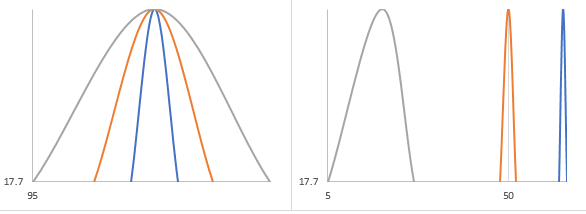Figure 4.2. Q-factor for several resonances. On the left, identical frequency with varying bandwidths. On the right, identical bandwidth with varying center frequencies (logarithmic axes).

The sharper the resonance, the quicker the resonance resonates during a sine sweep. The Q-factor measures the difference between a resonance that slowly ramps up during a sine sweep (a wide resonance with a low Q-factor) and a resonance that quickly ramps up during a sine sweep (a sharp resonance with a high Q-factor). The sharper the resonance, the harder it is to control. For an in-depth discussion on this topic, see the webinar on Sine Resonance Track & Dwell.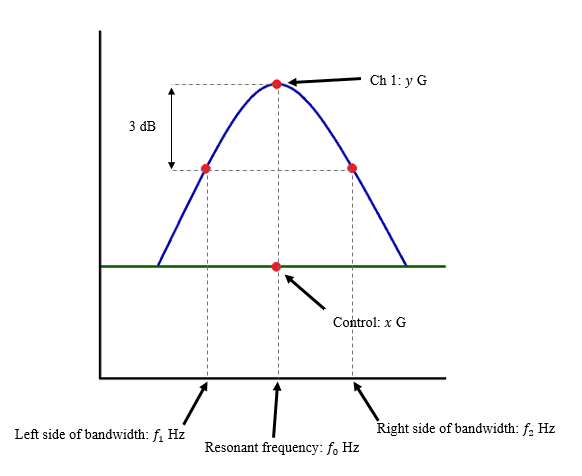(1)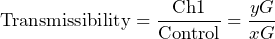(2)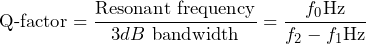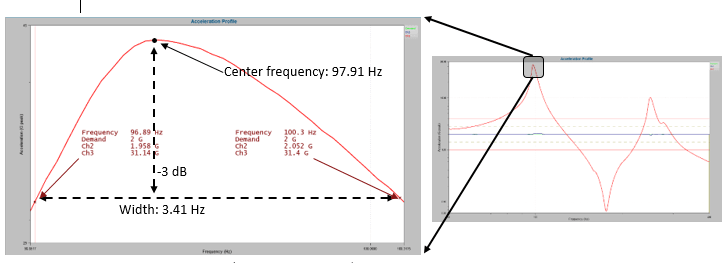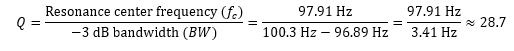Figure  4.3. Q-factor of a resonance (with some approximation).